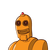# 5. From the choices given below, choose the equation whose graphs are given in Fig. 4.6and Fig. 4.7.For Fig. 4.6

5. From the choices given below, choose the equation whose graphs are given in Fig. 4.6
and Fig. 4.7.
For Fig. 4.6
For Fig. 4.7
(i) y = x
(i) y = x + 2
(ii) x + y = 0
(ii) y = x – 2
(iii) y = 2x
(iii) y = -x + 2
(iv) 2+ 3y = 7x
(iv) x+2y =6​

### 1 thought on “5. From the choices given below, choose the equation whose graphs are given in Fig. 4.6<br />and Fig. 4.7.<br />For Fig. 4.6<br />”

1.